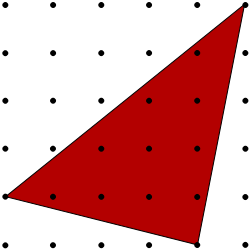#### You may also like### Kissing Triangles

Determine the total shaded area of the 'kissing triangles'.### Isosceles

Prove that a triangle with sides of length 5, 5 and 6 has the same area as a triangle with sides of length 5, 5 and 8. Find other pairs of non-congruent isosceles triangles which have equal areas.Four rods, two of length a and two of length b, are linked to form a kite. The linkage is moveable so that the angles change. What is the maximum area of the kite?

# Triangles in a Square

##### Age 11 to 14Challenge Level

These printable resources may be useful: Triangles in a SquareDotty Grid.

### Why do this problem?

This problem offers students a good opportunity to practise working out areas of triangles while using their visualisation skills to consider how to maximise and minimise the shaded area.

### Possible approach

You might like to hand out this worksheet, and some dotty paper.

Start by displaying the red triangle, and invite students to estimate its area, perhaps starting by asking whether they think that more or less than half of the dotty grid is covered.Once students have estimated the area, discuss how they might work out the area exactly. If no-one suggests it, invite them to consider subtracting the areas of the three unshaded triangles from the total area of the square grid.

Once everyone understands the method for working out the area of such triangles, invite them to find which of the four coloured triangles has the largest area. Again, they may wish to estimate the areas first.

Next, invite them to find all the possible areas of triangles with one vertex at (5,5), one on the left side of the grid and one on the bottom.

Finally, students could work towards an answer to the question "Can you find a general expression for the area of a triangle on this grid if its vertices have co-ordinates (5,5), (x,0) and (0,y)?"

### Key questions

Is it easier to work out the shaded area or the unshaded areas?
If I know the total area and the unshaded area, how can I work out the shaded area?
Is it possible to cover more than half the area of the grid with a triangle?

### Possible support

Isosceles Triangles offers practice in working out areas of triangles on a coordinate grid.

### Possible extension

Students could go on to explore the areas of triangles drawn on larger grids, or take a look at Quadrilaterals in a Square